# Introduction to Set – Data Structure and Algorithm Tutorials

## What is Set Data Structure?

In computer science, a set data structure is defined as a data structure that stores a collection of distinct elements.
It is a fundamental Data Structure that is used to store and manipulate a group of objects, where each object is unique. The Signature property of the set is that it doesn’t allow duplicate elements.

A set is a mathematical model for a collection of different things; a set contains elements or members, which can be mathematical objects of any kind numbers, symbols, points in space, lines, other geometrical shapes, variables, or even other sets.

A set can be implemented in various ways but the most common ways are:

• Hash-Based Set: the set is represented as a hash table where each element in the set is stored in a bucket based on its hash code.
• Tree-based set: In this implementation, the set is represented as a binary search tree where each node in the tree represents an element in the set.

## Need for Set Data Structure

Set data structures are commonly used in a variety of computer science applications, including algorithms, data analysis, and databases. The main advantage of using a set data structure is that it allows you to perform operations on a collection of elements in an efficient and organized way.

## Types of Set Data Structure:

The set data structure can be classified into the following two categories:

### 1. Unordered Set

An unordered set is an unordered associative container implemented using a hash table where keys are hashed into indices of a hash table so that the insertion is always randomized. All operations on the unordered set take constant time O(1) on an average which can go up to linear time O(n) in the worst case which depends on the internally used hash function, but practically they perform very well and generally provide a constant time lookup operation

### 2. Ordered Set

An Ordered set is the common set data structure we are familiar with. It is generally implemented using balanced BSTs and it supports O(log n) lookups, insertions and deletion operations.

## Set Data Structure in Different Languages:

### 1. Sets in C++

Sets in C++ internally implemented as (Self-Balancing Binary Search Tree)

Sets in C++ STL are a type of associative container in which each element has to be unique because the value of the element identifies it. The values are stored in a specific sorted order, i.e., ascending or descending.

The std::set class is the part of C++ Standard Template Library (STL) and it is defined inside the <set> header file.

Types of set in C++ STL:

Syntax:

`std::set <data_type> set_name;`

Datatype: The set can take any data type depending on the values, e.g. int, char, float, etc.

### 2. Sets in Java

Sets in Java internally implemented as (Hash-Table)

Set is an interface, objects cannot be created of the typeset. We always need a class that extends this list in order to create an object. And also, after the introduction of Generics in Java 1.5, it is possible to restrict the type of object that can be stored in the Set. This type-safe set can be defined as:

Types of set in Java:

Syntax:

```// Obj is the type of object to be stored in Set
Set<Obj> set = new HashSet<Obj> ();```

### 3. Sets in Python

Sets in Python internally implemented as (Hash-Table)

A Set in Python is an unordered collection data type that is iterable, mutable and has no duplicate elements.

Syntax:

`Set are represented by { } (values enclosed in curly braces)`

### 4. Sets in C#

Sets in C# internally implemented as (Hash-Table)

Set in C# is an unordered collection of unique elements. It comes under System.Collections.Generic namespace. It is used in a situation where we want to prevent duplicates from being inserted in the collection. As far as performance is concerned, it is better in comparison to the list.

Syntax:

` HashSet<int> set = new HashSet<int>();`

### 5. Sets in JavaScript

Sets in JavaScript internally implemented as (Hash-Table)

Set in JavaScript is a collection of items that are unique i.e. no element can be repeated. Set in ES6 are ordered: elements of the set can be iterated in the insertion order. A set can store any type of value whether primitive or objects.

Syntax:

`new Set([it]);`

Example:

array = [1,2,2,3,3,4,4,5] // Repeated values

Set = set(array)

SET(1,2,3,4,5) // only unique values

## Internal Implementation of Set Data Structure:

A set is a data structure that stores a collection of unique elements, with no duplicates allowed. Sets can be implemented using a variety of data structures, including arrays, linked lists, binary search trees, and hash tables.

Basically, a Set is language dependent Data Structure. Every language uses a different data structure to implement a set data structure internally like C++ uses Self-Balancing BST. Java, Python, C#, and JavaScript use Hash tables.

Sets in C++ use Self-Balancing Binary Tree(BST). In this approach, the elements are stored in nodes of a binary tree, with the property that the left subtree of any node contains only elements smaller than the node’s value, and the right subtree contains only elements larger than the node’s value. This property ensures that the elements in the tree are always sorted in ascending order.

• To add an element to the set implemented with a BST, you start at the root of the tree and compare the element to the node’s value.
• If the element is less than the node’s value, you traverse to the left subtree and repeat the process.
• If the element is greater than the node’s value, you traverse to the right subtree and repeat the process.
• If you reach a node with the same value as the element, you do not add the element to the tree since duplicates are not allowed.
• To remove an element from the set implemented with a BST, you first search for the node containing the element, following the same process as adding an element.
• If the element is not found in the tree, there is nothing to remove.
• If the element is found, there are three cases to consider:
• The node has no children: In this case, you simply remove the node from the tree.
• The node has one child: In this case, you replace the node with its child.
• The node has two children: In this case, you find the minimum element in the right subtree of the node (i.e., the leftmost node in the right subtree) and replace the node’s value with that element. Then, you remove the duplicate element from the right subtree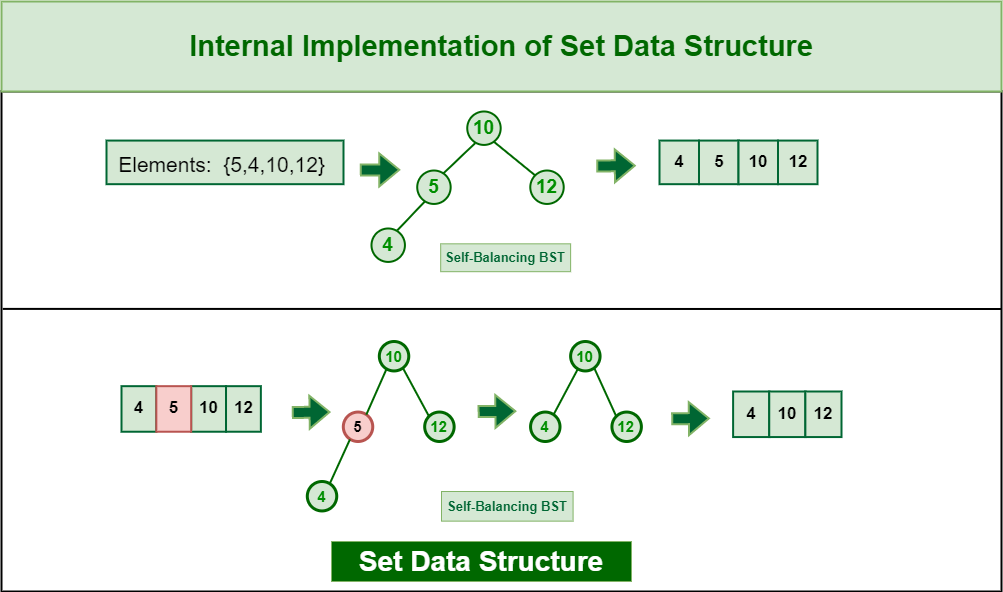Internal Implementation of Set Data Structure

In the case of implementation of Set using Hash table (as happens in Python) the implementation happens in the following way:

• To add an element to the set:
• Generate a hash value (say entry) for the key to be inserted.
• Go to the entry in the hash table and check if there are already other entries or not.
• If the slot is empty add the new element there.
• Otherwise, if there are other entries, traverse to the end of that entry list and add the new element there.
• To remove an element from the set:
• First, generate the hash value for the element.
• If the hash value is invalid or there are no entries then return an error message.
• Otherwise, go to that slot and find the element from the list present in that slot.
• Then remove that element from the hash table.

## Operations on Set Data Structure:

Here are some common operations that can be performed on a set data structure in C++ using the set container.

### 1. Insert an element:

You can insert an element into a set using the insert function. For example: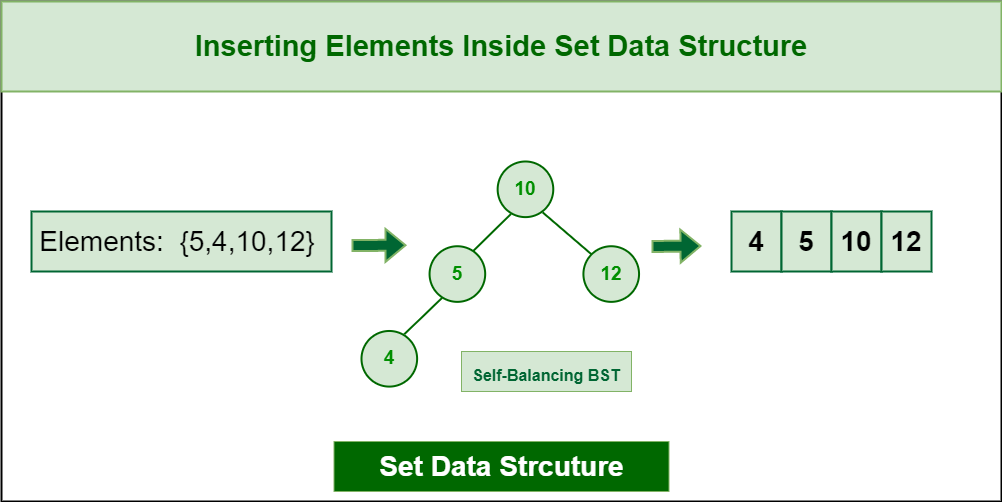Inserting Elements inside Set Data Structure

For hash table implementations it will be like the following: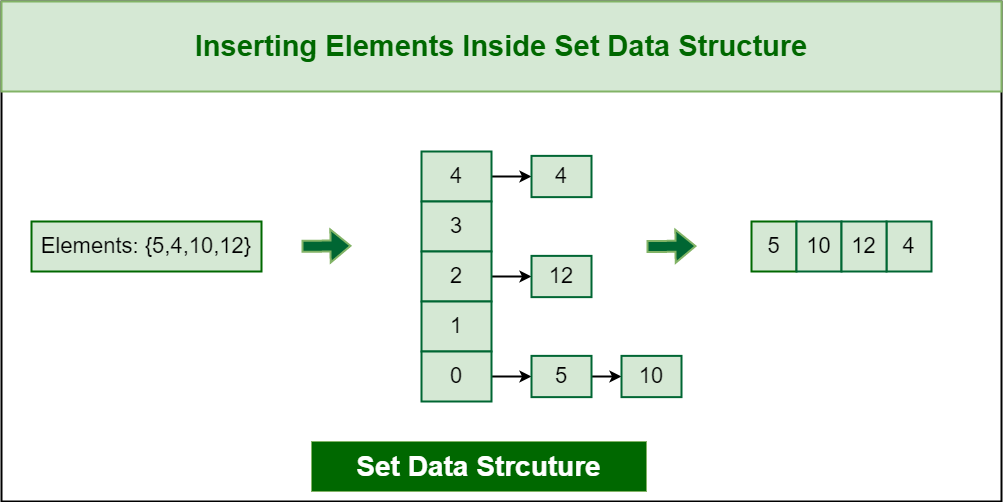Inserting Elements inside Set Data Structure

### 2. Check if an element is present:

You can check if an element is present in a set using the count function. The function returns 1 if the element is present, and 0 otherwise.

### 3. Remove an element:

You can remove an element from a set using the erase function. For example: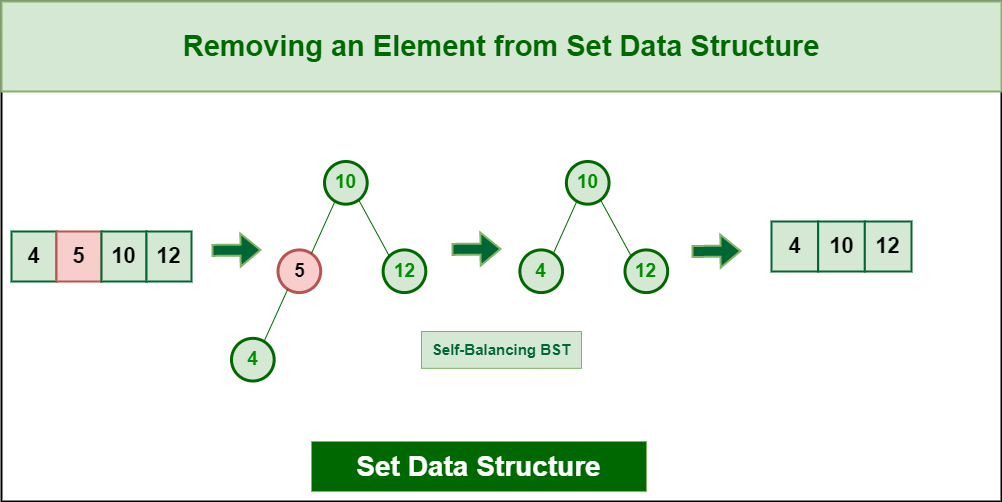Removing an Element from Set Data Structure

In the case of Hash table implementation it will be like the following: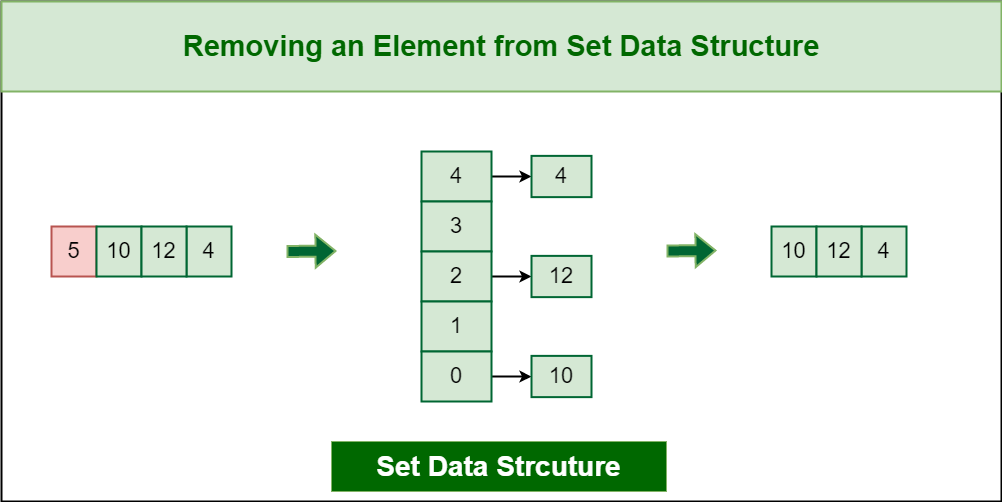Removing an Element from Set Data Structure

### 4. Find the minimum/maximum element:

You can find the minimum and maximum elements in a set using the begin and end iterators. The begin iterator points to the first element in the set, and the end iterator points to one past the last element.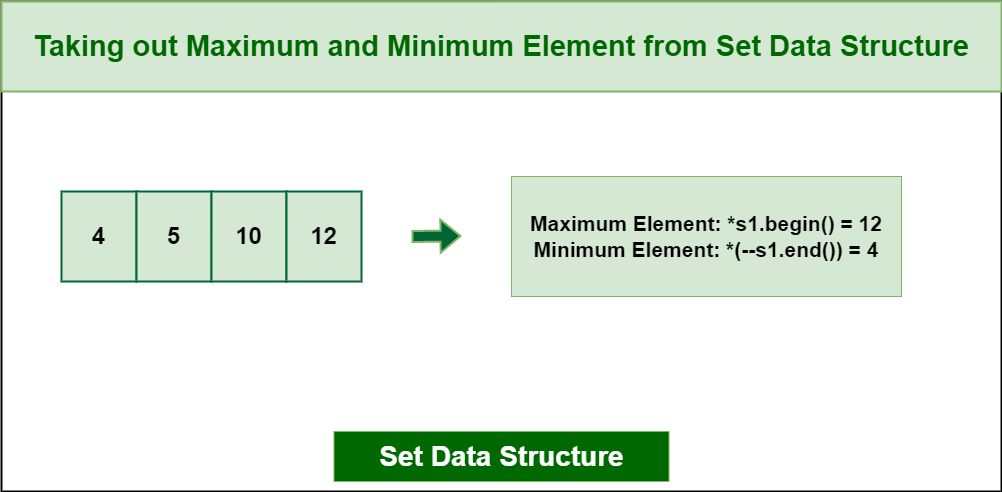Taking out Maximum and Minimum from Set Data Structure

In the case of hash table implementation in Python, the max() and min() functions return the maximum and the minimum respectively.

### 5. Get the size of the set:

You can get the size of a set using the size function.

## Implementation of Set Data Structure:

Below is the Implementation of the above operations:

## C++

 `#include ` `#include ` `using` `namespace` `std; ` ` `  `int` `main() ` `{ ` ` `  `    ``set<``int``> s1; ``// Declaring set ` ` `  `    ``// inserting elements in set ` `    ``s1.insert(10); ` `    ``s1.insert(5); ` `    ``s1.insert(12); ` `    ``s1.insert(4); ` ` `  `    ``// printing elements of set ` `    ``for` `(``auto` `i : s1) { ` `        ``cout << i << ``' '``; ` `    ``} ` `    ``cout << endl; ` ` `  `    ``// check if 10 present inside the set ` `    ``if` `(s1.count(10) == 1) { ` `        ``cout << ``"Element is present in the set:"` `<< endl; ` `    ``} ` ` `  `    ``// erasing 10 from the set ` `    ``s1.erase(5); ` ` `  `    ``// printing element of set ` `    ``for` `(``auto` `it : s1) { ` `        ``cout << it << ``" "``; ` `    ``} ` `    ``cout << endl; ` ` `  `    ``cout << ``"Minimum element: "` `<< *s1.begin() ` `         ``<< endl; ``// Printing maximum element ` `    ``cout << ``"Maximum element: "` `<< *(--s1.end()) ` `         ``<< endl; ``// Printing minimum element ` ` `  `    ``cout << ``"Size of the set is: "` `<< s1.size() ` `         ``<< endl; ``// Printing the size of the set ` ` `  `    ``return` `0; ` `}`

## Java

 `// Java program Illustrating Set Interface ` ` `  `// Importing utility classes ` `import` `java.util.*; ` ` `  `// Main class ` `public` `class` `GFG { ` ` `  `    ``// Main driver method ` `    ``public` `static` `void` `main(String[] args) ` `    ``{ ` ` `  `        ``// Creating an object of Set and ` `        ``// declaring object of type String ` `        ``Set hs = ``new` `HashSet(); ` ` `  `        ``// Custom input elements ` `        ``hs.add(``10``); ` `        ``hs.add(``5``); ` `        ``hs.add(``12``); ` `        ``hs.add(``4``); ` ` `  `        ``// Print the Set object elements ` `        ``System.out.println(``"Set is "` `+ hs); ` ` `  `        ``// Declaring a string ` `        ``int` `check = ``10``; ` ` `  `        ``// Check if the above string exists in ` `        ``// the SortedSet or not ` `        ``// using contains() method ` `        ``System.out.println(``"Contains "` `+ check + ``" "` `                           ``+ hs.contains(check)); ` ` `  `        ``// Printing elements of HashSet object ` `        ``System.out.println(hs); ` ` `  `        ``// Removing custom element ` `        ``// using remove() method ` `        ``hs.remove(``5``); ` ` `  `        ``// Printing Set elements after removing an element ` `        ``// and printing updated Set elements ` `        ``System.out.println(``"After removing element "` `+ hs); ` ` `  `        ``// finding maximum element ` `        ``Object obj = Collections.max(hs); ` `        ``System.out.println(``"Maximum Element = "` `+ obj); ` ` `  `        ``// finding maximum element ` `        ``Object obj2 = Collections.min(hs); ` `        ``System.out.println(``"Maximum Element = "` `+ obj2); ` ` `  `        ``// Displaying the size of the Set ` `        ``System.out.println(``"The size of the set is: "` `                           ``+ hs.size()); ` `    ``} ` `}`

## Python3

 `# set of letters ` `GEEK ``=` `{``10``, ``5``, ``12``, ``4``} ` ` `  `# adding 's' ` `GEEK.add(``15``) ` `print``(``"Letters are:"``, GEEK) ` ` `  `# adding 's' again ` `GEEK.add(``10``) ` `print``(``"Letters are:"``, GEEK) ` ` `  `# check if set contain an element ` `print``(``5` `in` `GEEK) ` ` `  `# removing an element from set ` `GEEK.remove(``5``) ` `print``(GEEK) ` ` `  `# print max element of set ` `print``(``max``(GEEK)) ` ` `  `# print min element of set ` `print``(``min``(GEEK)) ` ` `  `# printing size of the set ` `print``(``len``(GEEK)) `

## C#

 `// C# program Illustrating Set Interface ` `using` `System; ` `using` `System.Collections.Generic; ` ` `  `public` `class` `GFG { ` `    ``public` `static` `void` `Main() ` `    ``{ ` `        ``HashSet<``int``> hs ` `            ``= ``new` `HashSet<``int``>(); ``// Declaring set ` ` `  `        ``// inserting elements in set ` `        ``hs.Add(10); ` `        ``hs.Add(5); ` `        ``hs.Add(12); ` `        ``hs.Add(4); ` ` `  `        ``// printing elements of set ` `        ``foreach``(``int` `element ``in` `hs) ` `        ``{ ` `            ``Console.Write(element + ``" "``); ` `        ``} ` `        ``Console.WriteLine(); ` ` `  `        ``// check if 10 present inside the set ` `        ``if` `(hs.Contains(10)) { ` `            ``Console.WriteLine( ` `                ``"Element is present in the HashSet"``); ` `        ``} ` ` `  `        ``// erasing 10 from the set ` `        ``hs.Remove(5); ` ` `  `        ``// printing element of set ` `        ``foreach``(``int` `element ``in` `hs) ` `        ``{ ` `            ``Console.Write(element + ``" "``); ` `        ``} ` `        ``Console.WriteLine(); ` ` `  `        ``int` `minValue = ``int``.MaxValue; ` `        ``int` `maxValue = ``int``.MinValue; ` ` `  `        ``foreach``(``int` `element ``in` `hs) ` `        ``{ ` `            ``if` `(element < minValue) { ` `                ``minValue = element; ` `            ``} ` ` `  `            ``if` `(element > maxValue) { ` `                ``maxValue = element; ` `            ``} ` `        ``} ` ` `  `        ``// Printing minimum element ` `        ``Console.WriteLine(``"Minimum element: "` `+ minValue); ` ` `  `        ``// Printing maximum element ` `        ``Console.WriteLine(``"Maximum element: "` `+ maxValue); ` ` `  `        ``// Printing the size of the set ` `        ``Console.WriteLine(``"Size of the HashSet: "` `                          ``+ hs.Count); ` `    ``} ` `} `

## Javascript

 `// This is the JavaScript code for the above code ` `const s1 = ``new` `Set(); ``// Declaring set ` ` `  `// inserting elements in set ` `s1.add(10); ` `s1.add(5); ` `s1.add(12); ` `s1.add(4); ` ` `  `// printing elements of set ` `for` `(const i of s1) { ` `    ``console.log(i); ` `} ` ` `  `// check if 10 present inside the set ` `if` `(s1.has(10)) { ` `    ``console.log(``"Element is present in the set:"``); ` `} ` ` `  `// erasing 10 from the set ` `s1.``delete``(5); ` ` `  `// printing element of set ` `for` `(const it of s1) { ` `    ``console.log(it); ` `} ` ` `  `console.log(``"Minimum element: "` `+ Math.min(...s1)); ` `console.log(``"Maximum element: "` `+ Math.max(...s1)); ` `console.log(``"Size of the set is: "` `+ s1.size); ``// Printing the size of the set ` `//This code is contributed by sarojmcy2e`

Output

```4 5 10 12
Element is present in the set:
4 10 12
Minimum element: 4
Maximum element: 12
Size of the set is: 3```

### Complexity Analysis of Operations on Set Data Structure:

• Insertion: O(log n), where n is the number of elements in the set.
This is because std::set is implemented as a balanced binary search tree, and inserting an element into a balanced binary search tree takes O(log n) time
• Searching: O(log n), where n is the number of elements in the set.
• Deletion: O(log n), where n is the number of elements in the set.
• Accessing the minimum/maximum element: O(1), because std::set stores elements in sorted order, and the minimum and maximum elements can be accessed directly using the begin and end iterators.
• Size of the set: O(1), because std::set stores the number of elements in a separate variable, and accessing this variable takes constant time.

## Some Basic Operations/Terminologies Associated with Set Data Structure: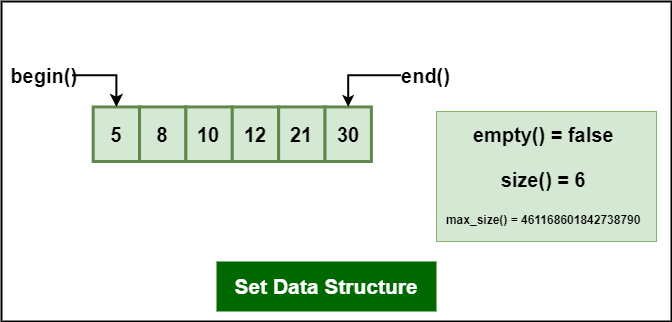Some Basic Operations/Terminologies Associated with Set Data Structure

• begin – Returns an iterator to the first element in the set.
• end – Returns an iterator to the theoretical element that follows the last element in the set.
• size – Returns the number of elements in the set.
• max_size – Returns the maximum number of elements that the set can hold.
• empty – Returns whether the set is empty.

Below is the Implementation of above Operations/Terminologies Associated with Set Data Structure:

## C++

 `// C++ program to demonstrate various functions of ` `// STL ` `#include ` `#include ` `#include ` `using` `namespace` `std; ` ` `  `int` `main() ` `{ ` `    ``// empty set container ` `    ``set<``int``, greater<``int``> > s1; ` ` `  `    ``// insert elements in random order ` `    ``s1.insert(40); ` `    ``s1.insert(30); ` `    ``s1.insert(60); ` `    ``s1.insert(20); ` `    ``s1.insert(50); ` ` `  `    ``// only one 50 will be added to the set ` `    ``s1.insert(50); ` `    ``s1.insert(10); ` ` `  `    ``// printing set s1 ` `    ``set<``int``, greater<``int``> >::iterator itr; ` `    ``cout << ``"\nThe set s1 is : \n"``; ` `    ``for` `(itr = s1.begin(); itr != s1.end(); itr++) { ` `        ``cout << *itr << ``" "``; ` `    ``} ` `    ``cout << endl; ` ` `  `    ``// assigning the elements from s1 to s2 ` `    ``set<``int``> s2(s1.begin(), s1.end()); ` ` `  `    ``// print all elements of the set s2 ` `    ``cout << ``"\nThe set s2 after assign from s1 is : \n"``; ` `    ``for` `(itr = s2.begin(); itr != s2.end(); itr++) { ` `        ``cout << *itr << ``" "``; ` `    ``} ` `    ``cout << endl; ` ` `  `    ``// remove all elements up to 30 in s2 ` `    ``cout << ``"\ns2 after removal of elements less than 30 "` `            ``":\n"``; ` `    ``s2.erase(s2.begin(), s2.find(30)); ` `    ``for` `(itr = s2.begin(); itr != s2.end(); itr++) { ` `        ``cout << *itr << ``" "``; ` `    ``} ` ` `  `    ``// remove element with value 50 in s2 ` `    ``int` `num; ` `    ``num = s2.erase(50); ` `    ``cout << ``"\ns2.erase(50) : "``; ` `    ``cout << num << ``" removed\n"``; ` `    ``for` `(itr = s2.begin(); itr != s2.end(); itr++) { ` `        ``cout << *itr << ``" "``; ` `    ``} ` ` `  `    ``cout << endl; ` ` `  `    ``// lower bound and upper bound for set s1 ` `    ``cout << ``"s1.lower_bound(40) : "` `<< *s1.lower_bound(40) ` `         ``<< endl; ` `    ``cout << ``"s1.upper_bound(40) : "` `<< *s1.upper_bound(40) ` `         ``<< endl; ` ` `  `    ``// lower bound and upper bound for set s2 ` `    ``cout << ``"s2.lower_bound(40) : "` `<< *s2.lower_bound(40) ` `         ``<< endl; ` `    ``cout << ``"s2.upper_bound(40) : "` `<< *s2.upper_bound(40) ` `         ``<< endl; ` ` `  `    ``return` `0; ` `}`

## Java

 `import` `java.util.*; ` ` `  `public` `class` `SetDemo { ` `    ``public` `static` `void` `main(String args[]) ` `    ``{ ` `        ``// Creating an empty Set ` `        ``Set set = ``new` `HashSet(); ` ` `  `        ``// Use add() method to add elements into the Set ` `        ``set.add(``1``); ` `        ``set.add(``2``); ` `        ``set.add(``3``); ` `        ``set.add(``4``); ` `        ``set.add(``5``); ` ` `  `        ``// Displaying the Set ` `        ``System.out.println(``"Set: "` `+ set); ` ` `  `        ``// Creating an iterator ` `        ``Iterator value = set.iterator(); ` ` `  `        ``// Displaying the values after iterating through the ` `        ``// iterator ` `        ``System.out.println(``"The iterator values are: "``); ` `        ``while` `(value.hasNext()) { ` `            ``System.out.println(value.next()); ` `        ``} ` `    ``} ` `}`

## Python3

 `# Python program to demonstrate various functions of set ` ` `  `# Creating an empty set ` `set` `=` `set``() ` ` `  `# Use add() method to add elements into the set ` `set``.add(``1``) ` `set``.add(``2``) ` `set``.add(``3``) ` `set``.add(``4``) ` `set``.add(``5``) ` ` `  `# Displaying the set ` `print``(``"Set:"``, ``set``) ` ` `  `# Creating an iterator ` `value ``=` `iter``(``set``) ` ` `  `# Displaying the values after iterating through the iterator ` `print``(``"The iterator values are:"``) ` `while` `True``: ` `    ``try``: ` `        ``print``(``next``(value)) ` `    ``except` `StopIteration: ` `        ``break`

## Javascript

 `// empty set container ` `let s1 = ``new` `Set(); ` ` `  `// insert elements in random order ` `s1.add(40); ` `s1.add(30); ` `s1.add(60); ` `s1.add(20); ` `s1.add(50); ` ` `  `// only one 50 will be added to the set ` `s1.add(50); ` `s1.add(10); ` ` `  `// printing set s1 ` `console.log(``"The set s1 is:"``); ` `for` `(let item of s1) { ` `    ``console.log(item); ` `} ` ` `  `// assigning the elements from s1 to s2 ` `let s2 = ``new` `Set(s1); ` ` `  `// print all elements of the set s2 ` `console.log(``"\nThe set s2 after assign from s1 is:"``); ` `for` `(let item of s2) { ` `    ``console.log(item); ` `} ` ` `  `// remove all elements up to 30 in s2 ` `console.log(``"\ns2 after removal of elements less than 30:"``); ` `for` `(let item of s2) { ` `    ``if` `(item < 30) { ` `        ``s2.``delete``(item); ` `    ``} ` `} ` `for` `(let item of s2) { ` `    ``console.log(item); ` `} ` ` `  `// remove element with value 50 in s2 ` `let num = s2.``delete``(50); ` `console.log(``"\ns2.delete(50): "` `+ num + ``" removed"``); ` `for` `(let item of s2) { ` `    ``console.log(item); ` `} ` ` `  `// lower bound and upper bound for set s1 ` `console.log(``"s1.has(40): "` `+ s1.has(40)); ` `console.log(``"s1.has(70): "` `+ s1.has(70)); ` ` `  `// lower bound and upper bound for set s2 ` `console.log(``"s2.has(40): "` `+ s2.has(40)); ` `console.log(``"s2.has(70): "` `+ s2.has(70)); `

## C#

 `using` `System; ` `using` `System.Collections.Generic; ` ` `  `class` `Program ` `{ ` `static` `void` `Main(``string``[] args) ` `{ ` `// Creating an empty set ` `HashSet<``int``> ``set` `= ``new` `HashSet<``int``>(); ` `    ``// Use Add() method to add elements into the set ` `    ``set``.Add(1); ` `    ``set``.Add(2); ` `    ``set``.Add(3); ` `    ``set``.Add(4); ` `    ``set``.Add(5); ` ` `  `    ``// Displaying the set ` `    ``Console.Write(``"Set: "``); ` `    ``foreach` `(``int` `element ``in` `set``) ` `    ``{ ` `        ``Console.Write(element + ``" "``); ` `    ``} ` `    ``Console.WriteLine(); ` ` `  `    ``// Creating an iterator ` `    ``IEnumerator<``int``> value = ``set``.GetEnumerator(); ` ` `  `    ``// Displaying the values after iterating through the iterator ` `    ``Console.WriteLine(``"The iterator values are:"``); ` `    ``while` `(value.MoveNext()) ` `    ``{ ` `        ``Console.WriteLine(value.Current); ` `    ``} ` `} ` `} `

Output

```The set s1 is :
60 50 40 30 20 10

The set s2 after assign from s1 is :
10 20 30 40 50 60

s2 after removal of elements less than 30 :
30 40 50 60
s2.erase(50) : 1 removed
30 40 60
s1.lower_bound(40) : 40
s1.upper_bound(40) : 30
s2.lower_bound(40) : 40
s2.upper_bound(40) : 60```

### Properties of Set Data Structure:

1. Storing order – The set stores the elements in sorted order.
2. Values Characteristics – All the elements in a set have unique values.
3. Values Nature – The value of the element cannot be modified once it is added to the set, though it is possible to remove and then add the modified value of that element. Thus, the values are immutable.
4. Search Technique – Sets follow the Binary search tree implementation.
5. Arranging order – The values in a set are unindexed.

### Applications of Set Data Structure:

Sets are abstract data types that can be used to store unique elements in a collection. Here are some common applications of sets:

• Removing duplicates: If you have a large collection of data, you can use a set to easily remove duplicates and store only unique elements.
• Membership testing: Sets provide efficient operations for checking if an element is a member of the set, which is useful in various algorithms and data structures.
• Set operations: Sets can be used to perform operations such as union, intersection, and difference, which are useful in mathematical set theory and computer science.
• Graph algorithms: Sets can be used to represent vertices and edges in graph algorithms, allowing for efficient operations such as finding connected components, detecting cycles, and computing minimum spanning trees.
• Cache replacement: Sets can be used to implement cache replacement policies, where the oldest or least recently used elements are removed when the cache is full.
• Database indexing: Sets can be used to implement indexes in databases, allowing for fast searching and retrieval of data based on specific keys or attributes.

### Advantages of Set Data Structure:

• Set can be used to store unique values in order to avoid duplications of elements present in the set.
• Elements in a set are stored in a sorted fashion which makes it efficient.
• Set is dynamic, so there is no error of overflowing of the set.
• Searching operation takes O(logN) time complexity.
• Sets provide fast and efficient operations for checking if an element is present in the set or not.
• Sets can be implemented using different data structures, such as HashSets and TreeSets, each with its own advantages and use cases.
• Sets can be used in a variety of applications, including algorithms, data analysis, and databases.
• Sets can be used to improve performance in many algorithms by providing fast lookups.

### Disadvantages of Set Data Structure:

• Elements in a set can only be accessed with pointers, there is no indexing in a set like arrays.
• Set is very complex to implement because of its structure and properties.
• A set takes O(logN) time complexity for basic operations like insertion and deletion.
• Not suitable for large data sets.
• Sets can only store elements of a specific data type.
• Sets can use more memory than other data structures, such as arrays or lists because they store each element in a separate location.

### Some Standard Problems Associated with Set Data Structure:

In conclusion, sets are a good choice for algorithms that require unique elements, fast searching, and sorting, but they may not be the best choice for algorithms that require fast insertions, allow duplicates, or have memory constraints. The choice of data structure should depend on the specific requirements of the algorithm.

Feeling lost in the world of random DSA topics, wasting time without progress? It's time for a change! Join our DSA course, where we'll guide you on an exciting journey to master DSA efficiently and on schedule.
Ready to dive in? Explore our Free Demo Content and join our DSA course, trusted by over 100,000 geeks!

Previous
Next